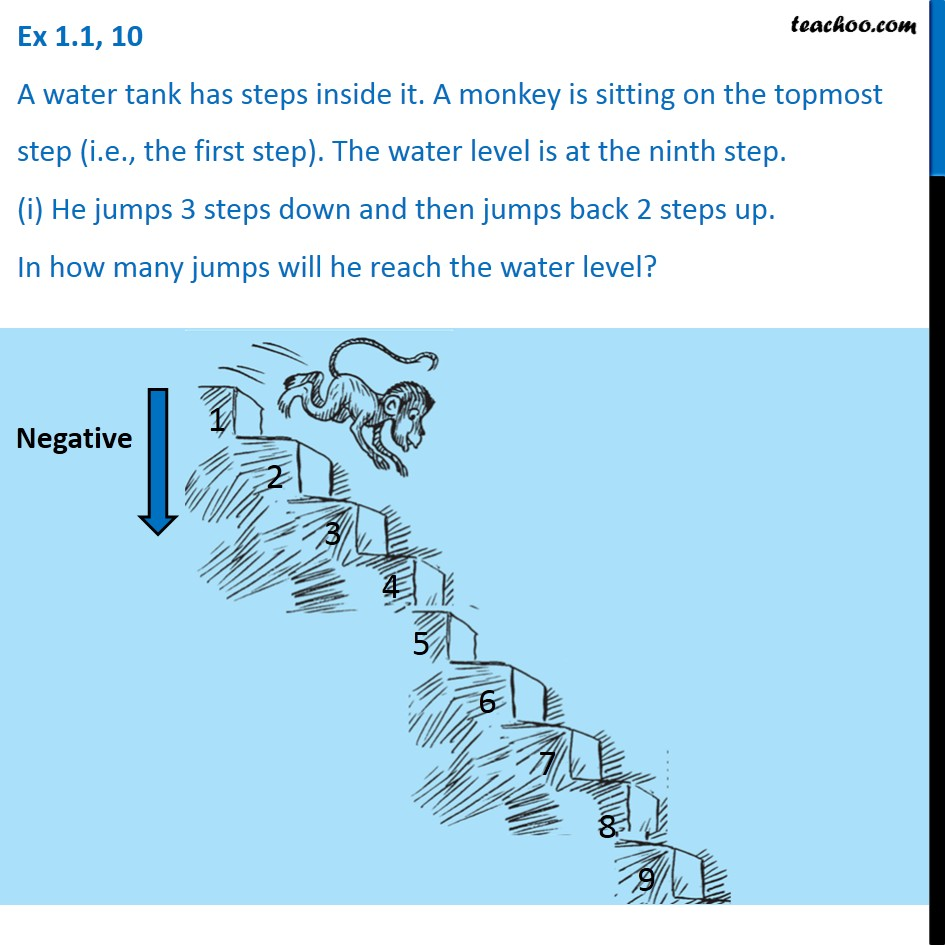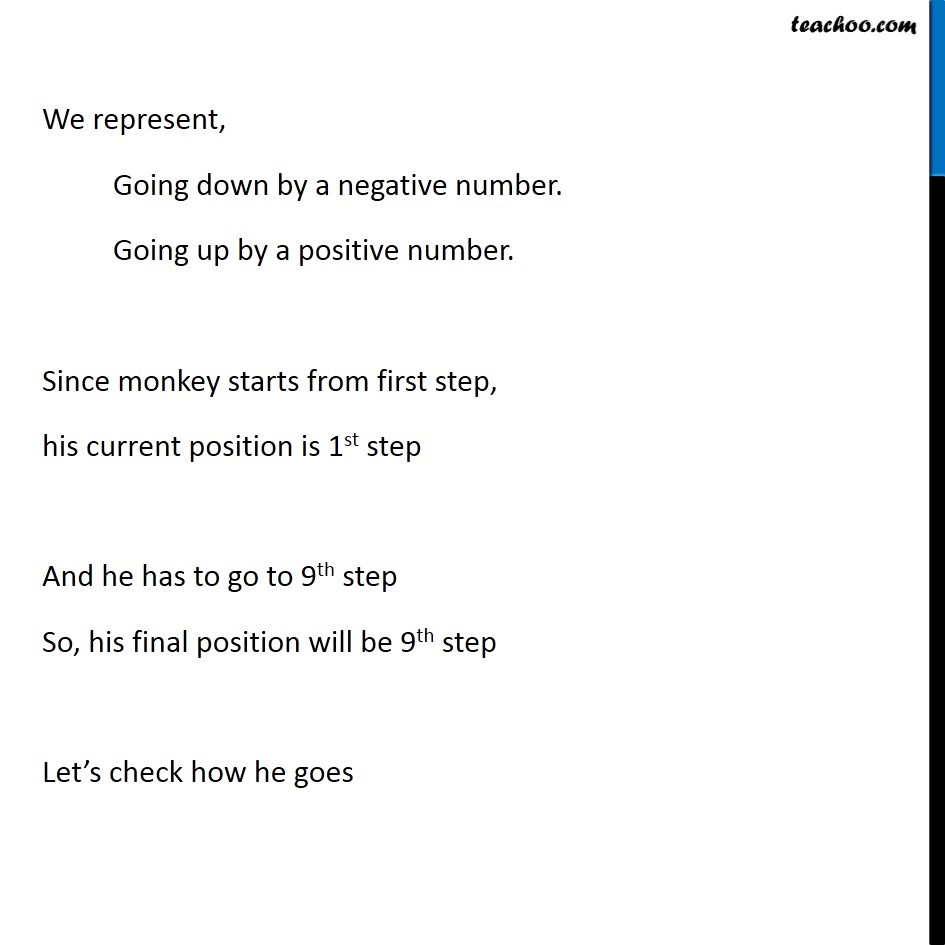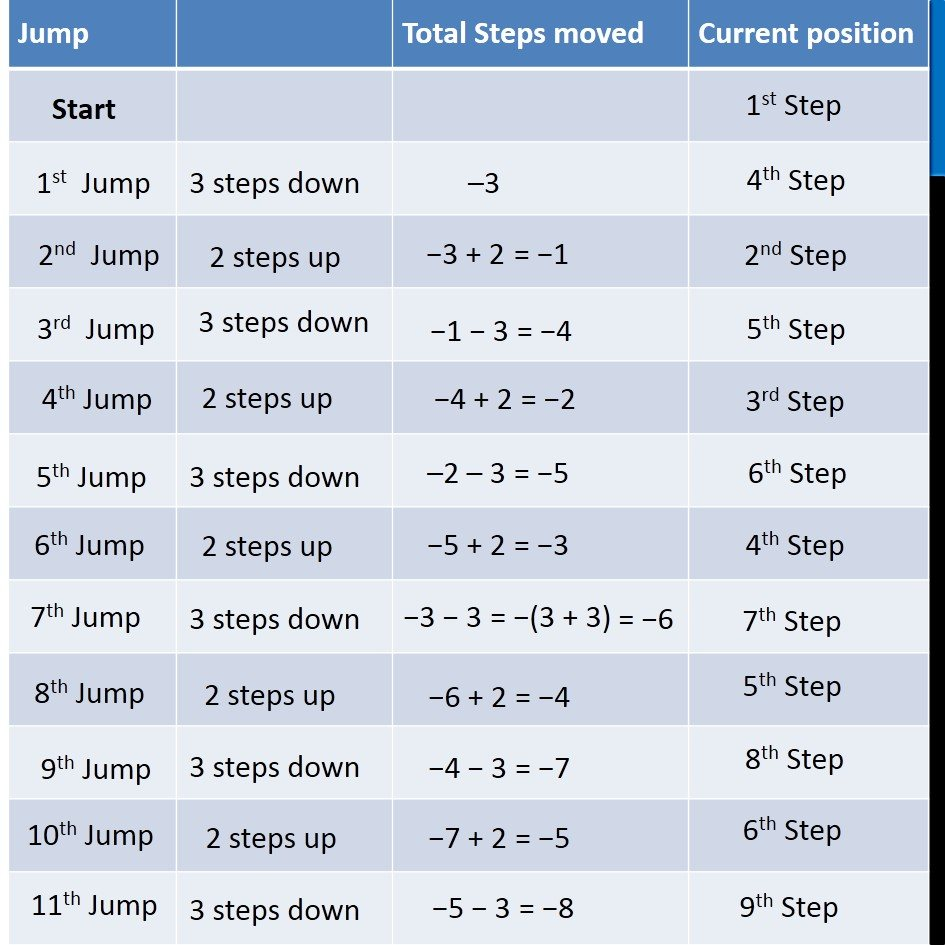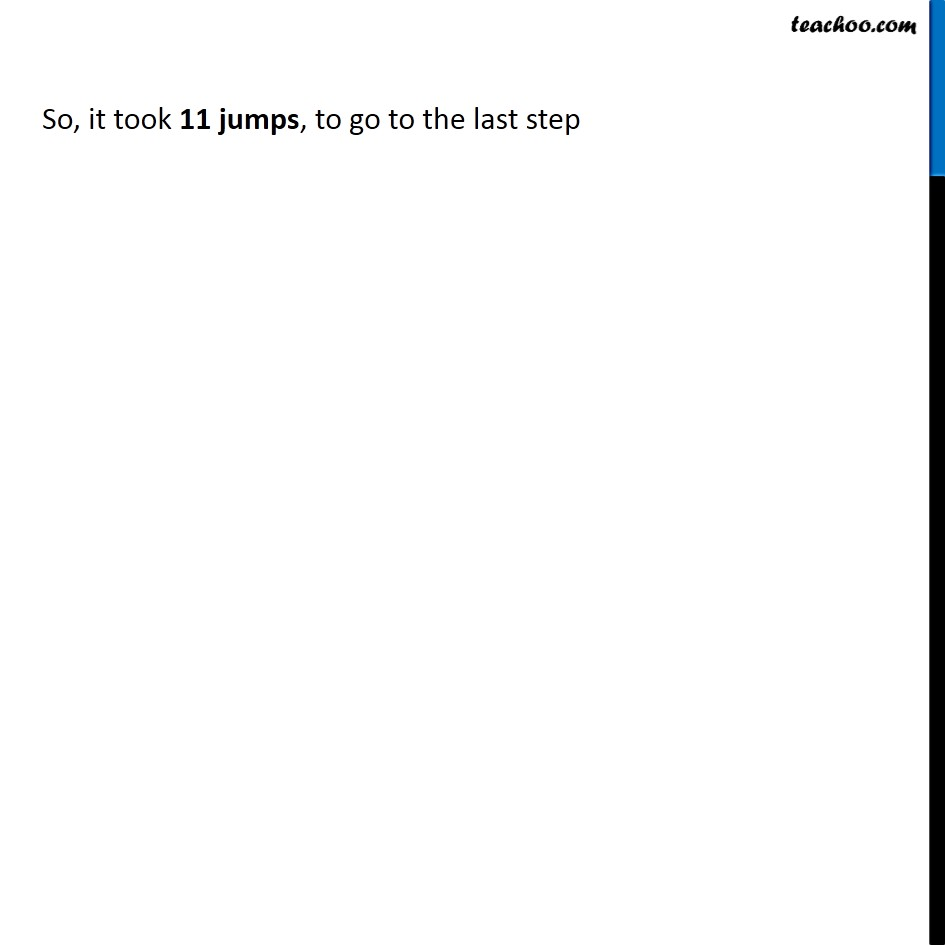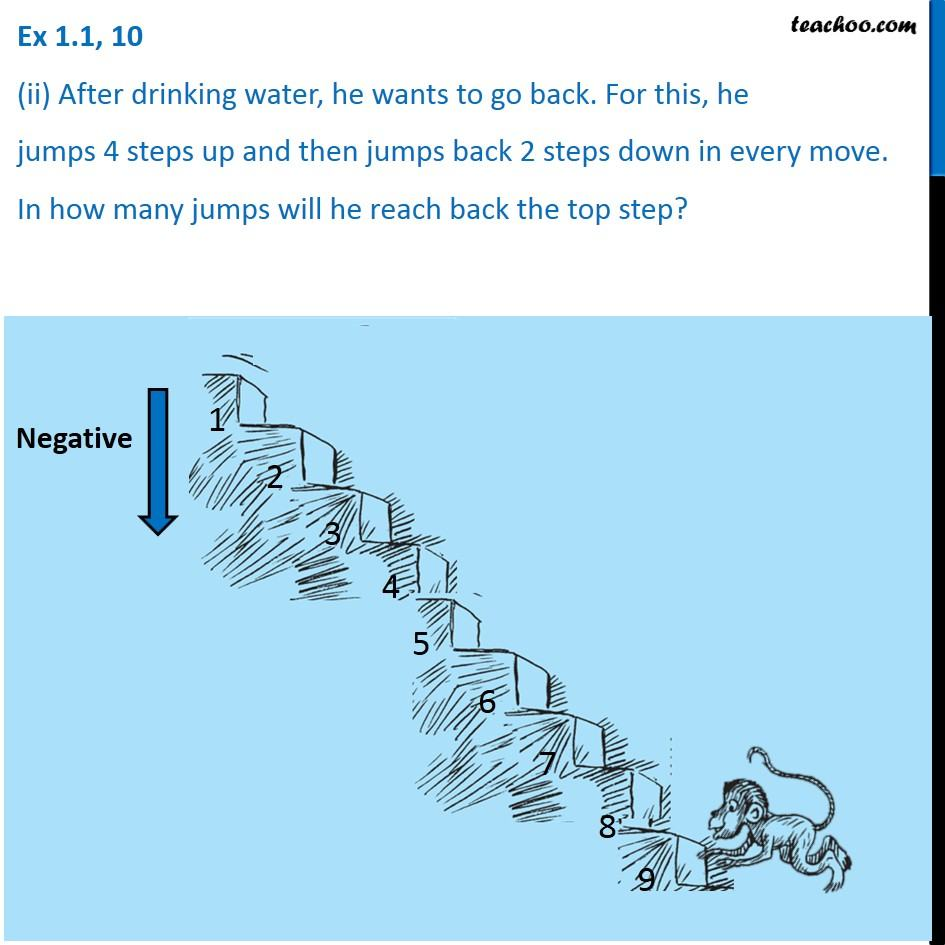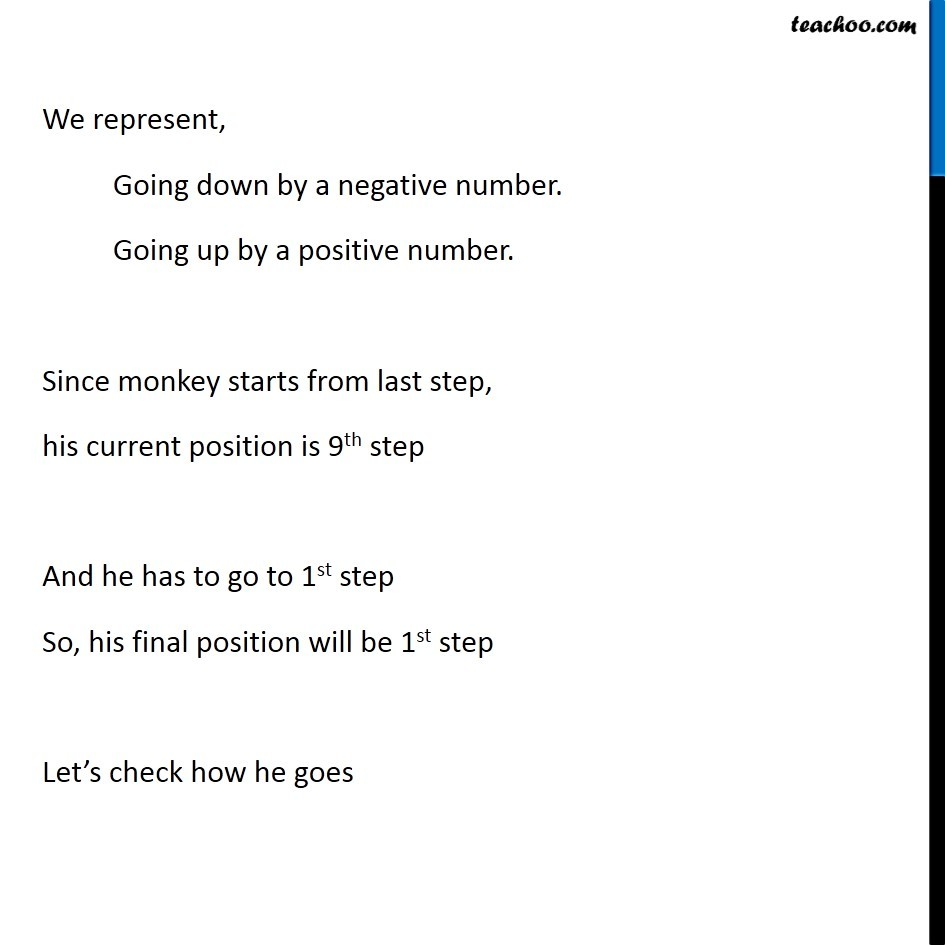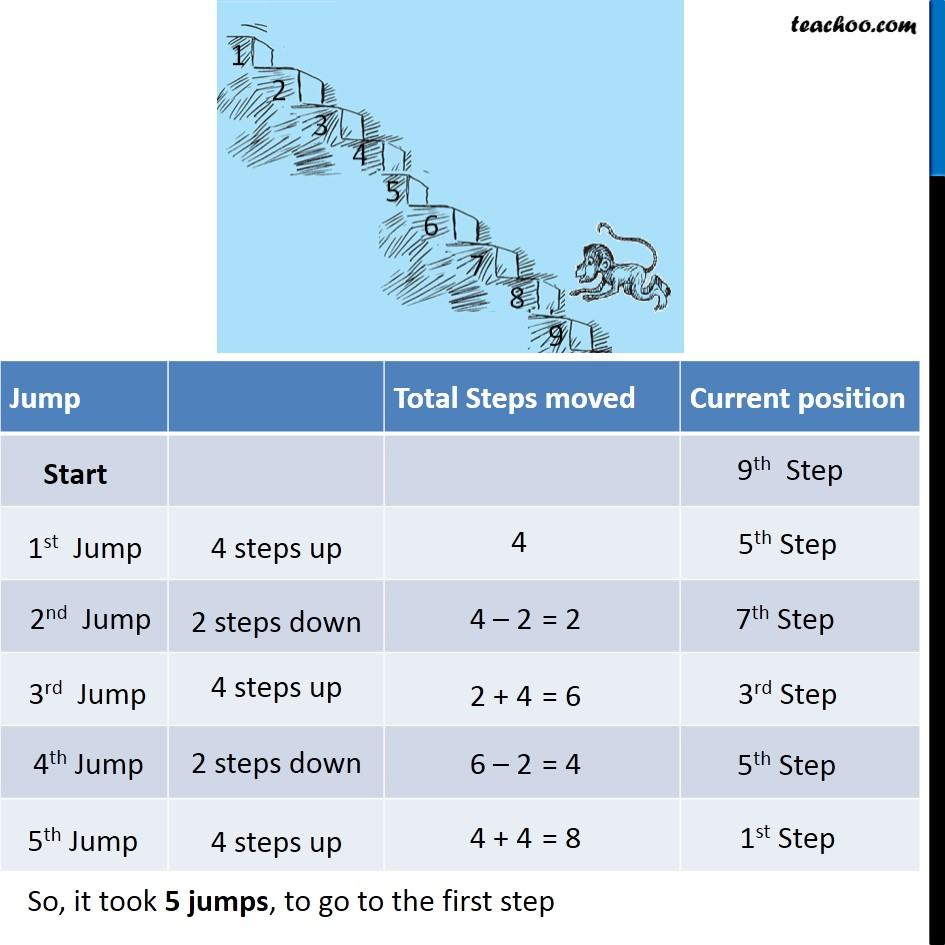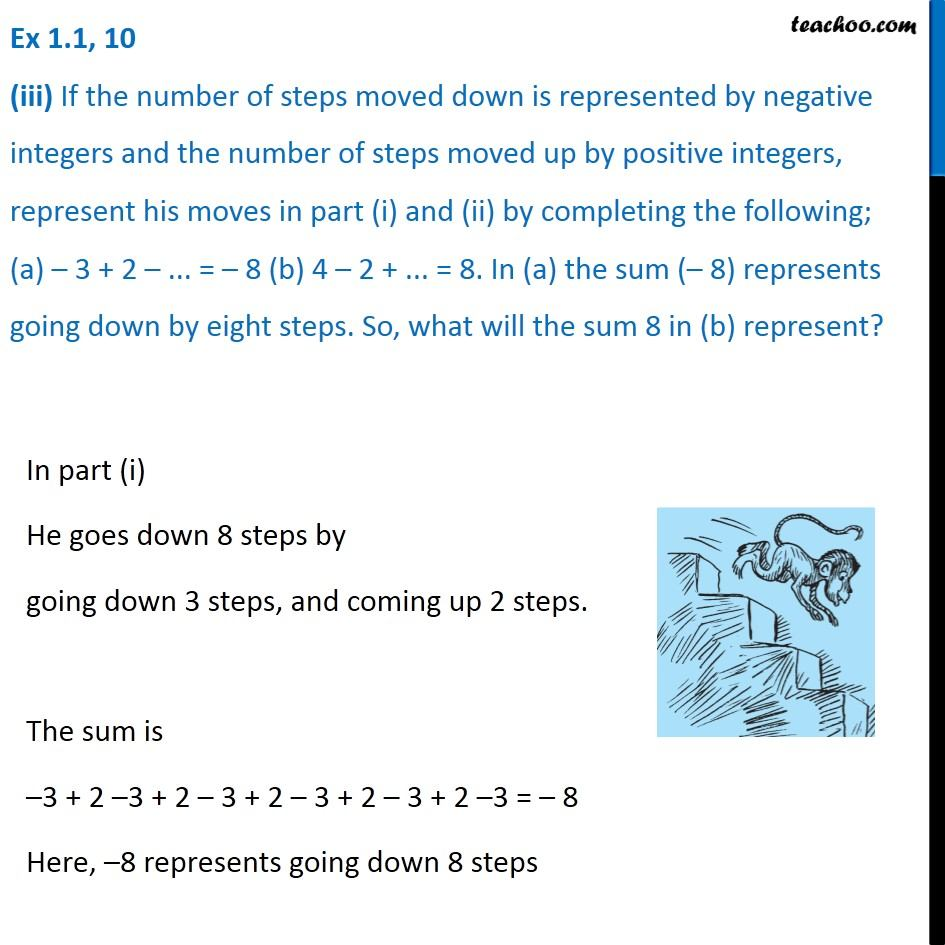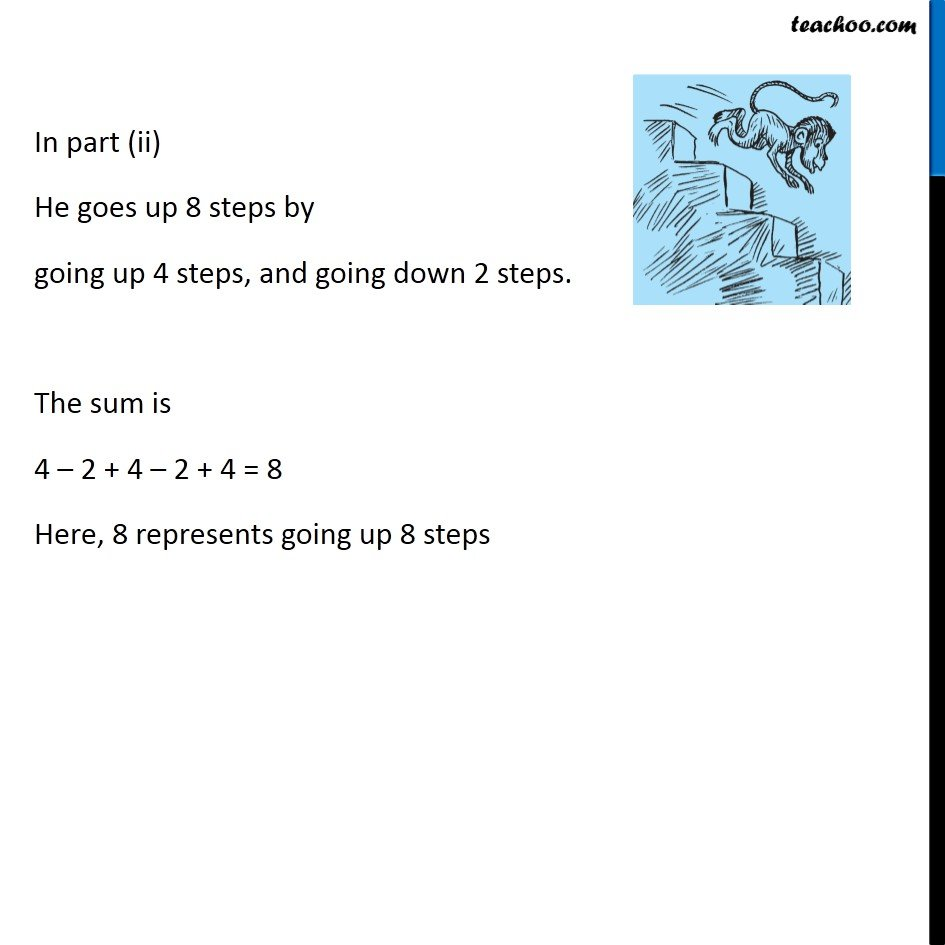1. Chapter 1 Class 7 Integers
2. Serial order wise
3. Ex 1.1

Transcript

Ex .1, 10 A water tank has steps inside it. A monkey is sitting on the topmost step (i.e., the first step). The water level is at the ninth step. (i) He jumps 3 steps down and then jumps back 2 steps up. In how many jumps will he reach the water level? Ex 1.1, 10 A water tank has steps inside it. A monkey is sitting on the topmost step (i.e., the first step). The water level is at the ninth step. (i) He jumps 3 steps down and then jumps back 2 steps up. In how many jumps will he reach the water level? We represent, Going down by a negative number. Going up by a positive number. Since monkey starts from first step, his current position is 1st step And he has to go to 9th step So, his final position will be 9th step Let’s check how he goes So, it took 11 jumps, to go to the last step Ex 1.1, 10 (ii) After drinking water, he wants to go back. For this, he jumps 4 steps up and then jumps back 2 steps down in every move. In how many jumps will he reach back the top step? We represent, Going down by a negative number. Going up by a positive number. Since monkey starts from last step, his current position is 9th step And he has to go to 1st step So, his final position will be 1st step Let’s check how he goes Ex 1.1, 10 (iii) If the number of steps moved down is represented by negative integers and the number of steps moved up by positive integers, represent his moves in part (i) and (ii) by completing the following; (a) – 3 + 2 – ... = – 8 (b) 4 – 2 + ... = 8. In (a) the sum (– 8) represents going down by eight steps. So, what will the sum 8 in (b) represent? In part (i) He goes down 8 steps by going down 3 steps, and coming up 2 steps. The sum is –3 + 2 –3 + 2 – 3 + 2 – 3 + 2 – 3 + 2 –3 = – 8 Here, –8 represents going down 8 steps In part (ii) He goes up 8 steps by going up 4 steps, and going down 2 steps. The sum is 4 – 2 + 4 – 2 + 4 = 8 Here, 8 represents going up 8 steps

Ex 1.1

Chapter 1 Class 7 Integers
Serial order wise